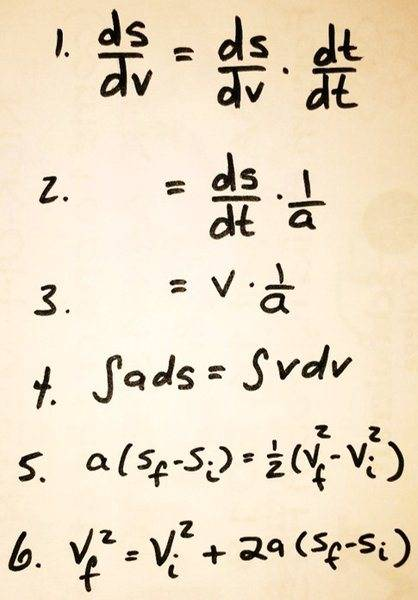# Kinematics derivation: what's "ds/dv" ?

• Taulant Sholla

#### Taulant Sholla

Hello, here's a derivation for one of the equations for Uniform Accelerated Motion (UAM). I like it because it's far more concise than the algebra version. Question though: is there any kind of meaningful interpretation of ds/dv (which starts everything off in step 1)? Thank you!#### Attachments

The derivation is usually done as follows: $$a=\frac{dv}{dt}=\frac{dv}{ds}\frac{ds}{dt}=v\frac{dv}{ds}$$where dv/ds is the rate of change of speed with respect to distance. So ds/dv is the reciprocal of dv/ds, but it doesn't have much fundamental significance.
•